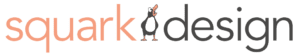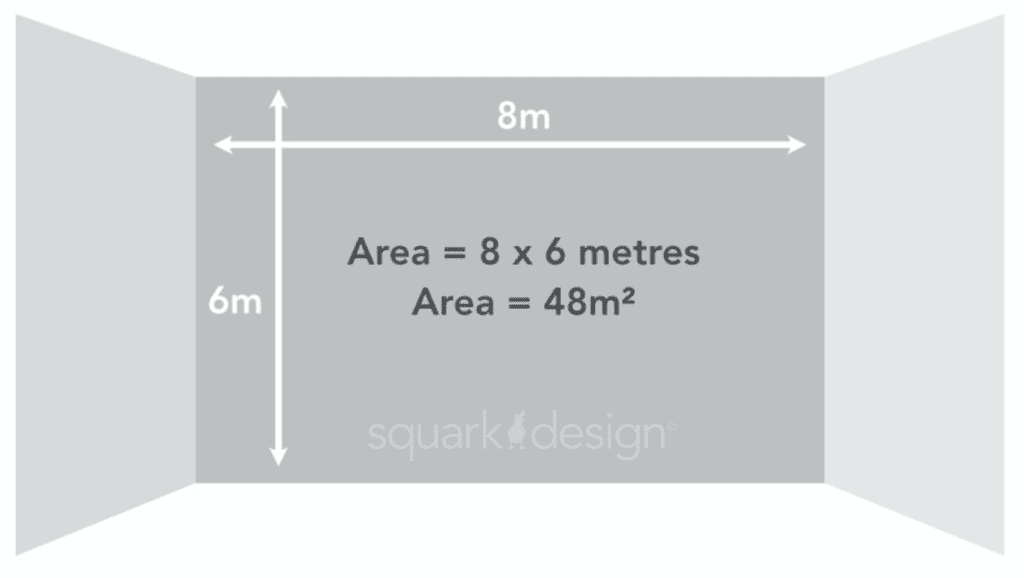e: info@squarkdesign.co.uk    t: 01476 245052

# How do I correctly work out the square meter of my walls?When measuring an area that you want to be covered in a material such as a bespoke wallpaper, you’re going to have to work out the number of square metres to figure out how much material you need to order.

### What’s the formula to calculate square metres

The formula is quite simple it is the length multiplied by the width together to get the area of a wall in square metres.

### How to calculate square metres?

Generally the space that you would be calculating the square metres for would be a flat square surface, if it is not square then you would need to try and break the total area down by different square sections and working out each total square metre by adding each of the calculated square metre blocks together, to measure out the area you will definitely need a tape measure handy.

To calculate square metre you will need to use a measuring tape and find out the length and width of the room.

### What unit should I measure in?

Measure them in centimetres or meters.

### Is there a difference between metres squared and square metres?

Yes, there is a big difference between metres squared and square metres. Square metres is calculated by length multiplied by width whereas metres squared has the same length and width.

### Inspiration Thursday

Sending you helpful inspiration and ideas once a week.

### When you are ready, get in touch